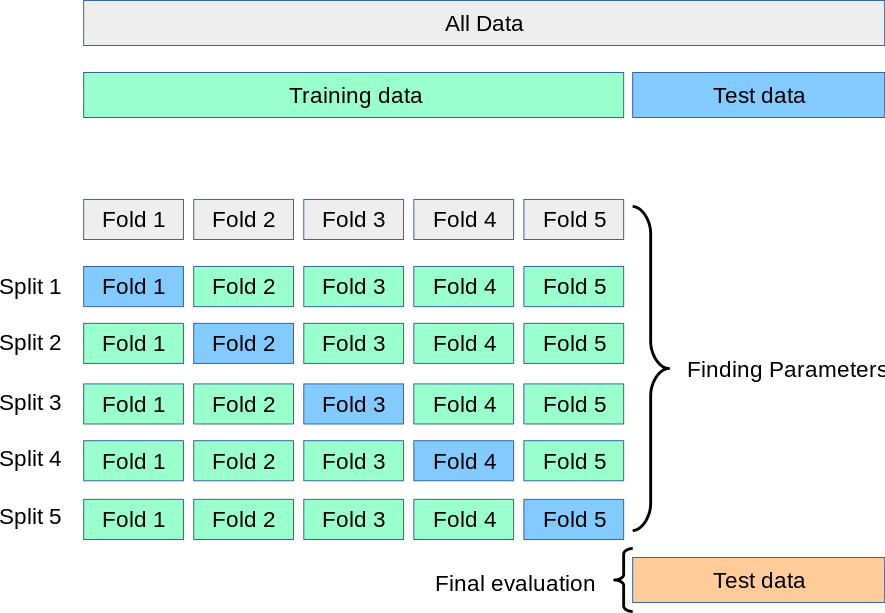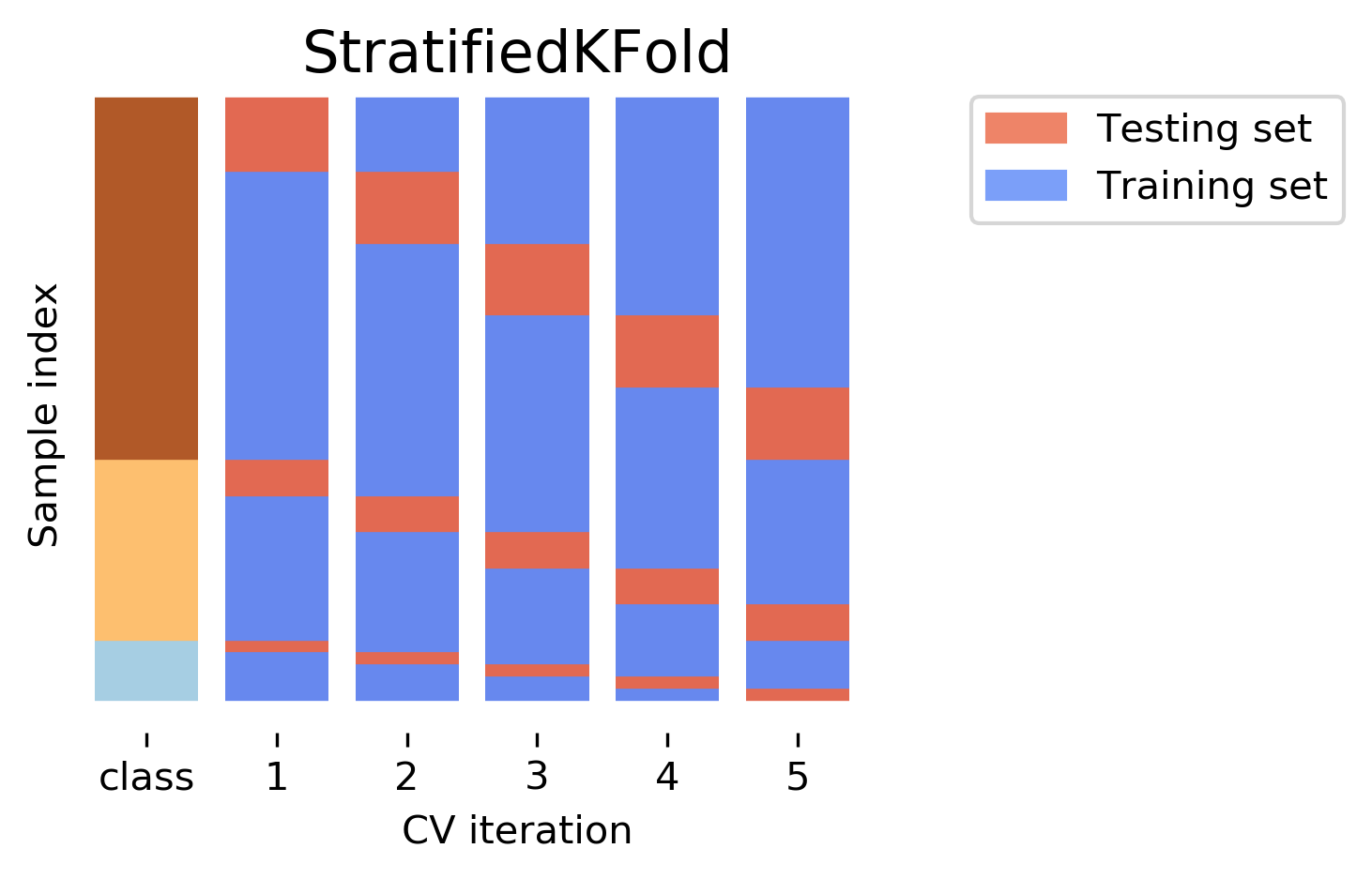# Understanding Cross-Validation: A Key Concept in Machine Learning

In today's world of data-driven decision-making, machine learning has emerged as a powerful tool for solving complex problems. One crucial technique in the toolkit of any machine learning practitioner is cross-validation. This blog post aims to provide a comprehensive introduction to cross-validation, including its types, the steps involved, and its benefits.

## What is Cross Validation?

In the realm of machine learning, the primary goal is to build models that perform well not just on the training data they've seen, but on unseen data as well. To evaluate this generalizability, we need a reliable method. This is where cross-validation comes into play.

Cross-validation is a statistical method used to estimate the performance of machine learning models. It helps us understand how the results of a statistical analysis will generalize to an independent dataset. It is primarily used in settings where the goal is prediction and one wants to estimate how accurately a predictive model will perform in practice.

It involves dividing the available data into multiple non-overlapping folds or subsets, using one of these folds as a validation set, and training the model on the remaining folds. This process is repeated multiple times, each time using a different fold as the validation set. Finally, the results from each validation step are averaged to produce a more robust estimate of the model’s performance.

The main purpose of cross validation is to prevent overfitting, which occurs when a model is trained too well on the training data and performs poorly on new, unseen data. By evaluating the model on multiple validation sets, cross validation provides a more realistic estimate of the model’s generalization performance, i.e., its ability to perform well on new, unseen data.

## Types of Cross Validation

Several forms of cross-validation are used in the field of machine learning, each with its own benefits and drawbacks. Some of the most common types include:

### K-Fold Cross Validation

K-fold cross validation is one of the most common and widely used cross validation techniques. It involves splitting the data into k equal-sized subsets or folds. One of these folds is used as the validation set, and the model is trained on the remaining k-1 folds. This process is repeated k times, each time using a different fold as the validation set. The performance of the model is then calculated by averaging the scores obtained on each validation set.If you look to the above image, you have devided your whole dataset into 5 folds. For the first iteration, you pick up the fold-1 to validate the model and the rest folds are used for trainig. Then in for the second iteration, you pick up the fold-2 (you should not to pick the fold-1 as it is already in the previous iteration) as testing dataset and the rest of the folds are used for training. This iterations are done for k times.

The advantage of k-fold cross validation is that it reduces the variance of the model performance estimate, as it uses all the data for both training and testing. The disadvantage is that it can be computationally expensive, especially for large datasets or complex models.

The choice of k depends on various factors, such as the size of the data, the complexity of the model, and the desired trade-off between bias and variance. A common value for k is 10, but it can vary depending on the situation. Below is the example to do the Cross Validation by making 10 folds using Scikit Learn library.

from sklearn.linear_model import LinearRegression
from sklearn.model_selection import cross_val_score

# Create linear regression model
model = LinearRegression()

# Perform 10-fold cross validation
scores = cross_val_score(model, X, y, cv=10)

# Print scores and mean score
print(scores)
print(scores.mean())


### Leave One Out Cross Validation (LOOCV)

Leave-one-out cross validation (LOOCV) is a special case of k-fold cross validation where k equals the number of observations in the data. In other words, it involves leaving out one observation from the data as the validation set, and training the model on the remaining n-1 observations. This process is repeated n times, each time using a different observation as the validation set. The performance of the model is then calculated by averaging the scores obtained on each validation set.The advantage of LOOCV is that it uses all the data for both training and testing, and it does not introduce any randomness in the data splitting. The disadvantage is that it can be very computationally expensive, especially for large datasets or complex models.

LOOCV is usually recommended when the data is very small or scarce, and every observation is valuable for training and testing. The following code snippet shows how to perform LOOCV on a linear regression model using scikit-leave:

from sklearn.linear_model import LinearRegression
from sklearn.model_selection import LeaveOneOut

# Create linear regression model
model = LinearRegression()

# Create leave-one-out splitter
loo = LeaveOneOut()

# Perform leave-one-out cross validation
scores = []
for train_index, test_index in loo.split(X):
X_train, X_test = X[train_index], X[test_index]
y_train, y_test = y[train_index], y[test_index]
model.fit(X_train, y_train)
score = model.score(X_test, y_test)
scores.append(score)

# Print scores and mean score
print(scores)
print(sum(scores)/len(scores))


### Stratified Cross Validation

Stratified cross validation is a variation of k-fold cross validation that preserves the proportion of each class or category in the data. It is useful when the data is imbalanced, i.e., when some classes or categories are overrepresented or underrepresented in the data. In such cases, using regular k-fold cross validation can result in biased or inaccurate estimates of the model performance, as some folds may contain only or mostly examples of one class or category.Stratified cross validation ensures that each fold has the same or similar distribution of classes or categories as the original data. This way, the model is trained and tested on more representative samples of the data.

Stratified cross validation can be applied to both classification and regression problems, depending on whether the target variable is categorical or continuous. For classification problems, stratified k-fold cross validation can be performed using scikit-learn’s StratifiedKFold class. For regression problems, stratified k-fold cross validation can be performed using scikit-learn’s StratifiedShuffleSplit class.

The following code snippet shows how to perform stratified 10-fold cross validation on a logistic regression model using scikit-learn:

from sklearn.linear_model import LogisticRegression
from sklearn.model_selection import StratifiedKFold

# Create logistic regression model
model = LogisticRegression()

# Create stratified 10-fold splitter
skf = StratifiedKFold(n_splits=10)

# Perform stratified 10-fold cross validation
scores = []
for train_index, test_index in skf.split(X, y):
X_train, X_test = X[train_index], X[test_index]
y_train, y_test = y[train_index], y[test_index]
model.fit(X_train, y_train)
score = model.score(X_test, y_test)
scores.append(score)

# Print scores and mean score
print(scores)
print(sum(scores)/len(scores))


## Common steps for CV

1. Data splitting: Divide the entire dataset into k equal, non-overlapping subsets or "folds". If the dataset has n samples, each fold should contain roughly n/k samples.
2. Model training: For each unique group, take the group as a test data set and take the remaining groups as a training data set. Fit a model on the training set and evaluate it on the test set.
3. Model evaluation: Retain the evaluation score and discard the model. Scores may include accuracy, F1-score, precision, recall, or others depending on the task at hand.
4. Results aggregation: The result of cross-validation is often given as the mean of the model skill scores, and also the variance or standard deviation of the scores which can give an idea about the model's stability. For example, a low standard deviation suggests the model is stable, and a high standard deviation suggests the model's performance is sensitive to the specific folds used for training and validation.
5. Model selection: If you're comparing different models, choose the one with the highest mean score (and, ideally, the lowest variance).
6. Final model training: Once the model type and hyperparameters have been selected, use all the data to train the final model.

## Benefits of Cross Validation

The beauty of cross-validation lies in its simplicity and effectiveness. Here are some reasons why cross-validation is widely used:

1. Model performance: It provides a robust estimate of the model's performance on unseen data. By using different subsets for training and validation in each iteration, we essentially use all our data for both training and validation, just not at the same time.
2. Bias Variance trade-off: It strikes a good balance between bias and variance. With k-fold cross-validation, we aren't using too small a part of our data for validation (which might lead to a high bias), and also not too large a part (which might lead to high variance).
3. Overfitting prevention: It helps in identifying models that overfit the training data. Overfitting is when a model performs very well on training data but poorly on unseen data. Since cross-validation involves testing the model's performance on unseen data, overfit models tend to stand out due to their poor validation performance.
4. Hyperparameter tuning: It helps in tuning the hyperparameters of the model. By using cross-validation, we can search for the best hyperparameters that give the best performance on the validation set.
5. Model selection: Cross-validation can help in selecting the model which would perform the best on unseen data. By comparing the validation scores of different models, we can select the one that performs the best.

## What is the final model after doing CV for prediction?

A common source of confusion in cross-validation is about the final model that should be used for predictions on new, unseen data. It's important to remember that the purpose of cross-validation is not to create the final model, but to assess how the model's results will generalize to an independent data set, and to tune any hyperparameters.

When you're performing k-fold cross-validation, you are indeed training k different models on k different subsets of the data. But the objective is to average the performance of these models, not to pick one of them for your final model.

After you have evaluated the cross-validation score and tuned hyperparameters, you usually train your model again with these optimal hyperparameters, but now using the entire dataset. This final model, trained on all available data, is the one you use for future predictions.

This approach makes intuitive sense because you're using the most information (i.e., the entire dataset) to train your final model. The cross-validation process only provides guidance on how to make that final model as good as possible. It tells you how well your model should perform on unseen data and which hyperparameters should give you the best performance.

## Conclusion

In summary, cross-validation is a valuable tool in a machine learning practitioner's arsenal. It's simple yet effective, providing a robust estimate of a model's real-world performance. Moreover, it helps in avoiding overfitting, tuning hyperparameters, and model selection. Despite its computational cost, the value it provides in building reliable and robust machine learning models is undeniable. Whether you're a seasoned practitioner or just starting out in the field of machine learning, understanding and effectively using cross-validation is an essential skill to have.

The journey doesn't stop here though! Stay tuned to learn more about other techniques to fine-tune your machine learning models and to ensure they're ready to tackle real-world challenges.